### Home > A2C > Chapter Ch4 > Lesson 4.2.4 > Problem4-125

4-125.
1. A line passes through the points (0, 2) and (1, 0). 4-125 HW eTool (Desmos). Homework Help ✎

1. Find the slope of the line.

2. Find the slope of a line parallel to the given line.

3. Find the slope of a line perpendicular to the given line.

4. Find the product of the slopes.

5. Make a conjecture about the product of the slopes of any two perpendicular lines.

6. Test your conjecture by creating more examples.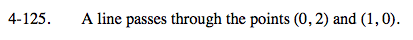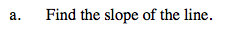Plot the points on a graph. Find the slope (rise over run).

$\textit{m}=\frac{\Delta\textit{y}}{\Delta\textit{x}}=\frac{2-0}{0-1}=-2$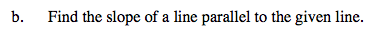Remember, parallel lines have the same slope.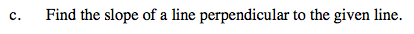Remember, perpendicular lines form a right angle.
The slopes will be the negative reciprocals of each other.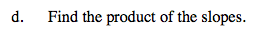Use the values from parts (b) and (c).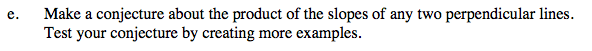The product of the slopes of two perpendicular lines is always the same number.

Use the eTool below to explore the line.
Click the link at right for the full version of the eTool: A2C 4-125 HW eTool